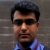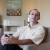# A Binomial Problem

Six Sigma – iSixSigma Forums General Forums General A Binomial Problem

• This topic has 6 replies, 2 voices, and was last updated 3 years, 11 months ago byRahul.
Viewing 7 posts - 1 through 7 (of 7 total)
• Author
Posts
• #55033Tracy
Guest

Can someone help to solve and explain how to solve this question

A sampling plan involves selecting a sample of 50 from a very large lot, and rejecting the lot if 2 or more defects are found. If an incoming lot is 10% defective, what is the probability of rejecting the lot?

A 0.6335
B 0.9987
C 0.5443
D 0.9662

0
#198288Amit Kumar Ojha
Participant

Hi,

Here is the solution….
First of all consider this as a problem of Binomial Distribution. Our objective is to find the probability of getting 2 or more defects (thats when the lot will be rejected).
Technically we can write it as P(X>=2)
It is given in the problem that X is no. of defects, n=50, p=0.1, q=0.9

P(X>=2) => 1- [P(X=0) + P(X=1)]

P(X=0) => 50C0*(0.1^0)*(0.9^50) = 0.00515
P(X=1) => 50C1*(0.1^1)*(0.9^49) = 0.02863

Hence P(X>=2) => 1 – (0.00515 + 0.02863)
=> 0.966214

Hence Option D is correct Answer

Please let me know if you need any further clarification on this or any other such calculations.

Good Luck!!!!

0
#198293Norbert Feher
Participant

Or in minitab:

1. Go to Graph/Probability distribution Plot!
2. Select view probability
3. Select Binomial distribution!
Number of trials:50
Probability:0,1
4. Select the shaded area tab
5. Select X value instead of probability
Right tail
X value:2

Result graph: shows a red shaded area of 0,9662

So the previous answer was right :-)

0
#198302Amit Kumar Ojha
Participant

Norbert shared a very simple way by which you can get the answer without getting into complexity of calculating the probabilities.

In the above problem, since the calculations were not so tedious, hence you can do either way. However, in many real life scenarios the calculations are too complicated to be done manually.

Hence it is good to use minitab, the way Norbert explained.

Thanks Norbert :-))

0
#198313Tracy
Guest

Hi,

Thank you all for helping me solve the problem. Amit Kumar Your explanation is very easy to understand. This problem is from a CQE practice exam.

I want to post the exam writer’s answer to this question. I had a hard time to understand his explanation although the answer is D.

“Since the probability of success (a defect) is constant on each trial, the binomial distribution can be used to compute this probability. The probability of no successes in 50 trials, with the probability of success on a single trial of 0.1 is 0.0052. The probability of 1 success in 50 trials, with the probability of success on a single trial of 0.1 is 0.0286. The probability of 2 or more defects is 1-0.0052-0.0286=0.9662.”

does the no successes means 0 defect?
and 1 success means exactly 1 defect?

what does the success on a single trial of 0.1 means?

0
#198331Amit Kumar Ojha
Participant

Yes Tracy.

You are right. No success in this case means 0 defects and 1 success implies 1 defect.
Success on single trial of 0.1 means that if you test a sample of 100 units what is the probability of getting 10 defects.

Hope I clarified your doubt :-))

Best of Luck!!!

0
#202623Rahul
Guest

Why are you using Binomial instead of Poisson?

0
Viewing 7 posts - 1 through 7 (of 7 total)

You must be logged in to reply to this topic.